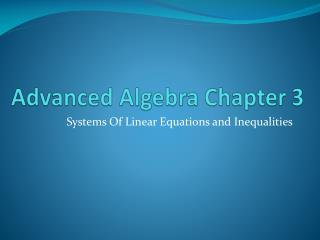DownloadDownload PresentationAdvanced Algebra Chapter 3

# Advanced Algebra Chapter 3

Download Presentation## Advanced Algebra Chapter 3

- - - - - - - - - - - - - - - - - - - - - - - - - - - E N D - - - - - - - - - - - - - - - - - - - - - - - - - - -
##### Presentation Transcript

1. Advanced Algebra Chapter 3 Systems Of Linear Equations and Inequalities

2. Solving Systems by Graphing—3.1

3. System of two linear equations: • Solution: The ordered pair (x, y) that satisfies both equations • Where the equations intersect

4. Check to see if the following point is a solution of the linear system: (2, 2)

5. Check to see if the following point is a solution of the linear system: (0, -1)

6. Solving Graphically

7. Solving Graphically

8. Solving Graphically

9. Solving Graphically

10. Interpretation • The graphs intersect at 1 specific point • Exactly one solution • The graph is a single line • Infinitely many solutions • The graphs never intersect • No solutions

11. p.142 #11-49 Odd

12. Solving Systems Algebraically—3.2

13. Substitution Method • 1.) Solve one of the equations for one of the variables • 2.) Substitute the expression into the other equation • 3.) Find the value of the variable • 4.) Use this value in either of the original equations to find the 2nd variable

14. Substitution Method

15. Substitution Method

16. Substitution Method

17. Substitution Method

18. p.152#11-19

19. Solving by Linear Combination • 1.) Multiply 1 or both equations by a constant to get similar coefficients • 2.) Add or subtract the revised equations to get 1 equation with only 1 variable • Something must cancel! • 3.) Solve for the variable • 4.) Use this value to solve for the 2nd variable • 5.) Smile 

20. Linear Combinations

21. Linear Combinations

22. Linear Combinations

23. Linear Combinations

24. p153 #23-31

25. Pop Quiz!!Graphing Linear Inequalities • Graph the following:

26. Graphing Linear Inequalities

27. Graphing Linear Inequalities

28. Graphing Linear Inequalities

29. Solving Systems of Linear Inequalities—3.3

30. Systems • Solution of two linear equations: • Ordered pair • Solution of two linear inequalities • Infinite Solutions • An entire region

31. Solving Linear Inequalities

32. Solving Linear Inequalities

33. Solving Linear Inequalities

34. Solving Linear Inequalities

35. p.159 #13-49 EOO

36. Optimization—3.4

37. Optimization • Optimization • Finding the maximum or minimum value of some quantity • Linear Programming: • Optimizing linear functions • Objective Function: • What we are trying to maximize or minimize • The linear inequalities making up the program: constraints • Points contained in the graph: feasible region

38. Optimal Solution • The optimal Solution (minimum or maximum value) must occur at a vertex of the feasible region • If the region is bounded, a minimum and maximum value must occur within the feasible region

39. Solving: Finding min and max • Objective Function: • Constraints:

40. Solving: Finding min and max • Objective Function: • Constraints:

41. Solving: Finding min and max • Objective Function: • Constraints:

42. A Furniture Manufacturer makes chairs and sofas from prepackaged parts. The table below gives the number of packages of wood parts, stuffing, and material required for each chair and sofa. The packages are delivered weekly and manufacturer has room to store 1300 packages of wood parts, 2000 packages of stuffing, and 800 packages of fabric. The manufacturer profits \$200 per chair and \$350 per sofa. How many of each should they make per week?

43. Writing Inequalities • Optimization: • Constraints:

44. p.166 #9-15, 21

45. Graphing in Three Dimensions—3.5

46. z-axis • Ordered triple • Octants

47. (-2, 1, 6)

48. (3, 4, 0)

49. (0, 4, -2)

50. Linear Equations ax + by + cz = d An ordered triple is a solution of the equation The graph of an equation of three variables is the graph of all it’s solutions -The graph will be a plane